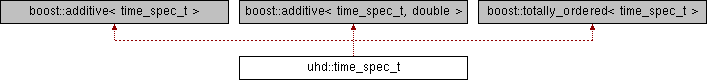USRP Hardware Driver and USRP Manual  Version: 3.14.0.0.rc1-70-g864d4002d UHD and USRP Manualuhd::time_spec_t Class Reference

`#include <uhd/types/time_spec.hpp>`

Inheritance diagram for uhd::time_spec_t:## Public Member Functions

time_spec_t (double secs=0)

time_spec_t (int64_t full_secs, double frac_secs=0)

time_spec_t (int64_t full_secs, long tick_count, double tick_rate)

long get_tick_count (double tick_rate) const

long long to_ticks (const double tick_rate) const

double get_real_secs (void) const

int64_t get_full_secs (void) const

double get_frac_secs (void) const

time_spec_toperator+= (const time_spec_t &)
Implement addable interface. More...

time_spec_toperator+= (double &)

time_spec_toperator-= (const time_spec_t &)
Implement subtractable interface. More...

time_spec_toperator-= (double &)

## Static Public Member Functions

static time_spec_t from_ticks (long long ticks, double tick_rate)

## Detailed Description

A time_spec_t holds a seconds and a fractional seconds time value. Depending upon usage, the time_spec_t can represent absolute times, relative times, or time differences (between absolute times).

The time_spec_t provides clock-domain independent time storage, but can convert fractional seconds to/from clock-domain specific units.

The fractional seconds are stored as double precision floating point. This gives the fractional seconds enough precision to unambiguously specify a clock-tick/sample-count up to rates of several petahertz.

## ◆ time_spec_t() [1/3]

 uhd::time_spec_t::time_spec_t ( double secs = `0` )

Create a time_spec_t from a real-valued seconds count.

Parameters
 secs the real-valued seconds count (default = 0)

## ◆ time_spec_t() [2/3]

 uhd::time_spec_t::time_spec_t ( int64_t full_secs, double frac_secs = `0` )

Create a time_spec_t from whole and fractional seconds.

Parameters
 full_secs the whole/integer seconds count frac_secs the fractional seconds count (default = 0)

## ◆ time_spec_t() [3/3]

 uhd::time_spec_t::time_spec_t ( int64_t full_secs, long tick_count, double tick_rate )

Create a time_spec_t from whole seconds and fractional ticks. Translation from clock-domain specific units.

Parameters
 full_secs the whole/integer seconds count tick_count the fractional seconds tick count tick_rate the number of ticks per second

## ◆ from_ticks()

 static time_spec_t uhd::time_spec_t::from_ticks ( long long ticks, double tick_rate )
static

Create a time_spec_t from a 64-bit tick count. Translation from clock-domain specific units.

Parameters
 ticks an integer count of ticks tick_rate the number of ticks per second

## ◆ get_frac_secs()

 UHD_INLINE double uhd::time_spec_t::get_frac_secs ( void ) const

Get the fractional part of the time in seconds.

Returns
the fractional seconds

## ◆ get_full_secs()

 UHD_INLINE int64_t uhd::time_spec_t::get_full_secs ( void ) const

Get the whole/integer part of the time in seconds.

Returns
the whole/integer seconds

## ◆ get_real_secs()

 double uhd::time_spec_t::get_real_secs ( void ) const

Get the time as a real-valued seconds count. Note: If this time_spec_t represents an absolute time, the precision of the fractional seconds may be lost.

Returns
the real-valued seconds

## ◆ get_tick_count()

 long uhd::time_spec_t::get_tick_count ( double tick_rate ) const

Convert the fractional seconds to clock ticks. Translation into clock-domain specific units.

Parameters
 tick_rate the number of ticks per second
Returns
the fractional seconds tick count

## ◆ operator+=() [1/2]

 time_spec_t& uhd::time_spec_t::operator+= ( const time_spec_t & )

## ◆ operator+=() [2/2]

 time_spec_t& uhd::time_spec_t::operator+= ( double & )

## ◆ operator-=() [1/2]

 time_spec_t& uhd::time_spec_t::operator-= ( const time_spec_t & )

Implement subtractable interface.

## ◆ operator-=() [2/2]

 time_spec_t& uhd::time_spec_t::operator-= ( double & )

## ◆ to_ticks()

 long long uhd::time_spec_t::to_ticks ( const double tick_rate ) const

Convert the time spec into a 64-bit tick count. Translation into clock-domain specific units.

Parameters
 tick_rate the number of ticks per second
Returns
an integer number of ticks

The documentation for this class was generated from the following file: